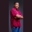Related Tags

scala
area
circle
communitycreator

# How to calculate the area of a circle in ScalaTheodore Kelechukwu Onyejiaku

### Overview

The area of a circle is the region occupied by the circle. It is the product of pi (π) and the square of the circle’s radius. To do this, we need to create a constant variable pi that will hold our pi value. Then we multiply this value by the square of the radius. We need to use the Math.pow() method to calculate the squared value of the radius. To do this, we need to import the scala.math library.

### Syntax

pi * Math.pow(radius, 2)
Syntax for finding the area of a circle in Scala

### Parameters

pi: This is the pi constant variable.

radius: This is the given radius of the circle.

### Return value

A float value is returned, which is the area of the circle.

### Example

// import math package
import scala.math._

object Main extends App {
// create a constant
val PI = 3.14F

// get area of circles
var area1 = PI * Math.pow(radius1, 2)
var area2 = PI * Math.pow(radius2, 2)
var area3 = PI * Math.pow(radius3, 2)
var area4 = PI * Math.pow(radius4, 2)

// print values
println(area1)
println(area2)
println(area3)
println(area4)
}
Get the area of a circle in Scala

### Explanation

• Line 2: We import the math module.
• Line 6: We create a PI value.
• Line 9–12: We create some radii.
• Line 15–18: We get the area of some circles.
• Line 21–24: We print the areas to the console.

RELATED TAGS

scala
area
circle
communitycreator

CONTRIBUTORTheodore Kelechukwu Onyejiaku
RELATED COURSES

View all Courses

Keep Exploring

Learn in-demand tech skills in half the time